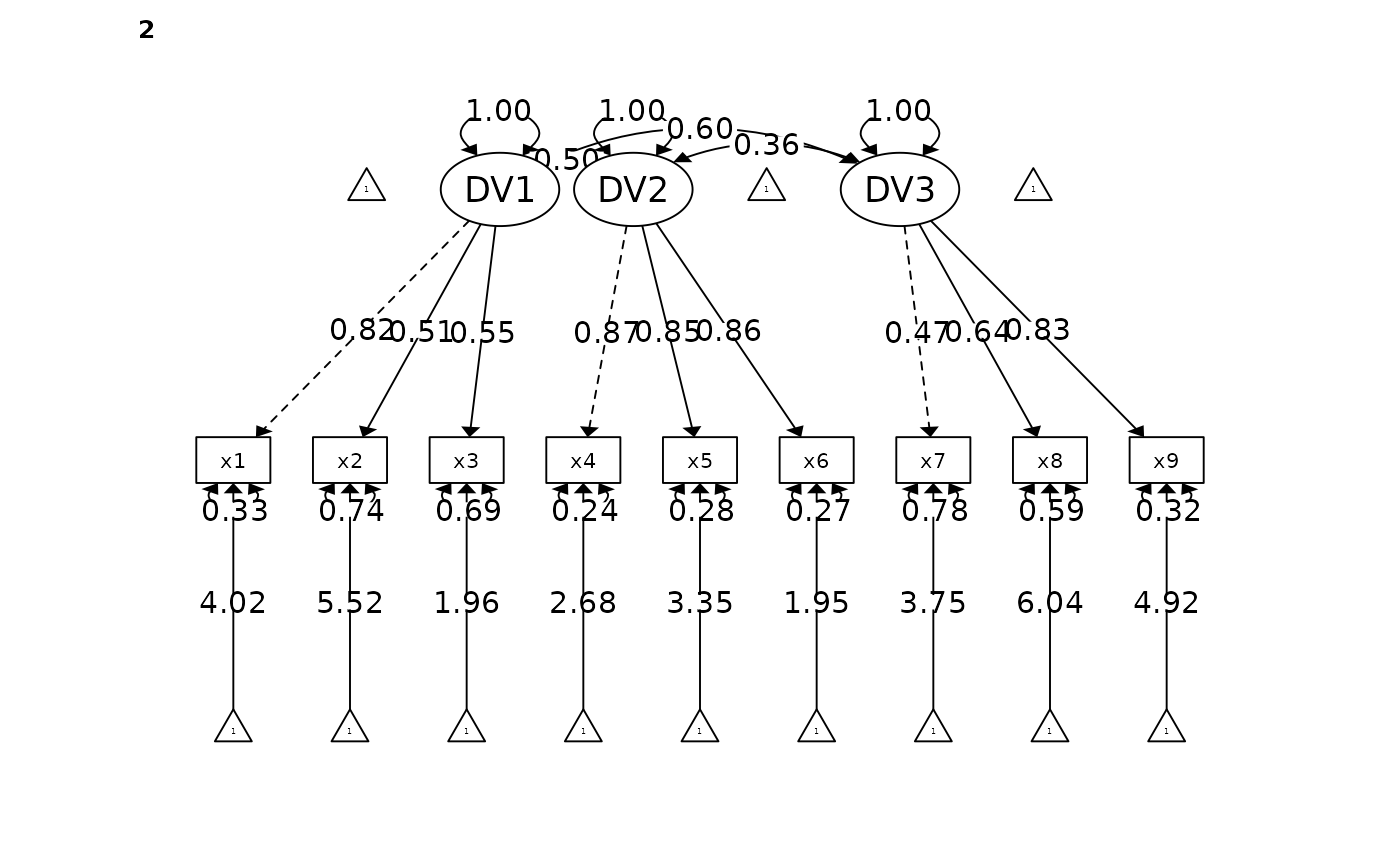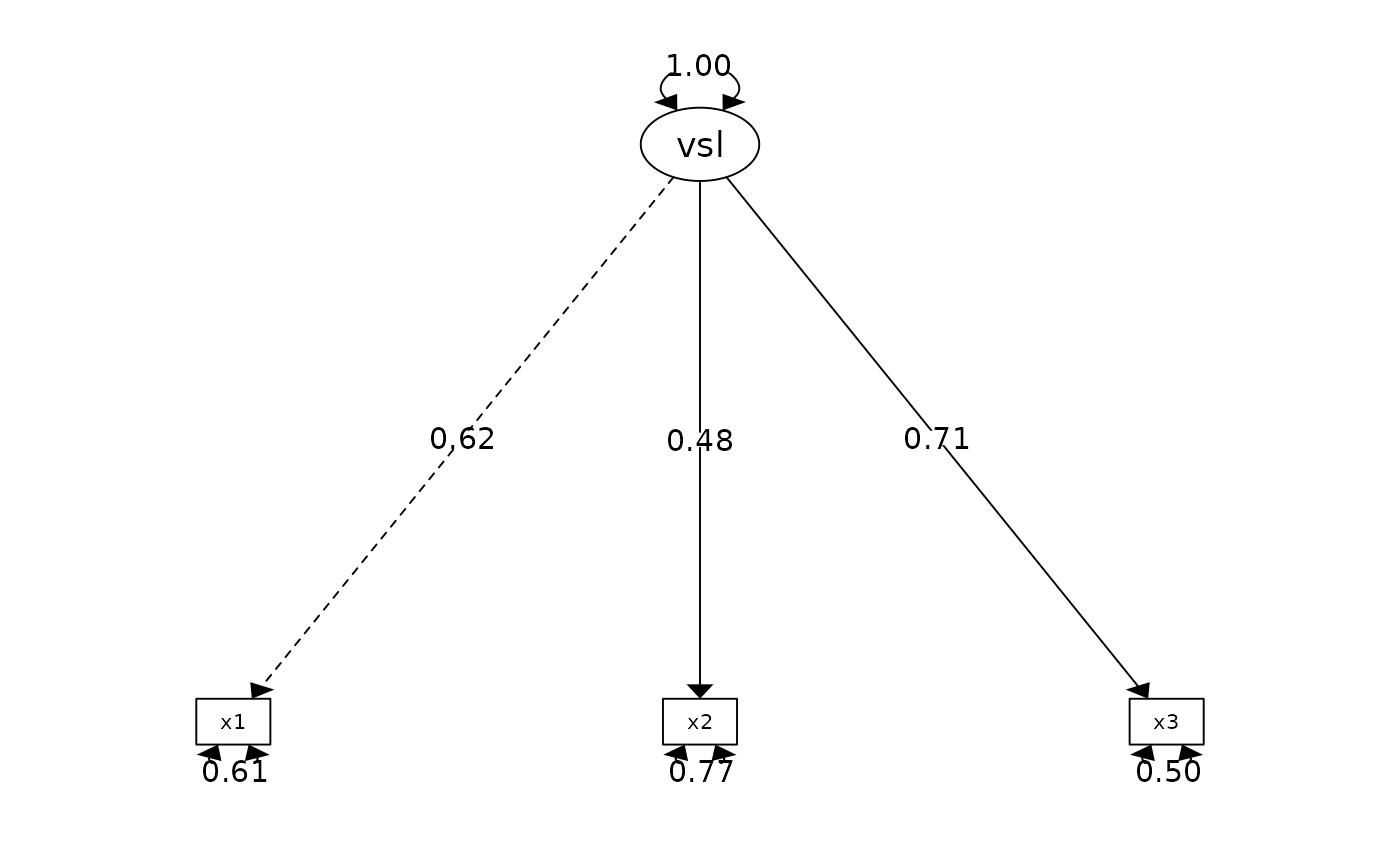The function fits a CFA model using the lavaan::cfa(). Users can fit single and multiple factors CFA, and it also supports multilevel CFA (by specifying the group). Users can fit the model by passing the items using dplyr::select() syntax or an explicit lavaan model for more versatile usage. All arguments (except the CFA items) must be explicitly named (e.g., model = your-model; see example for inappropriate behavior).

cfa_summary(
data,
...,
model = NULL,
group = NULL,
ordered = FALSE,
digits = 3,
model_covariance = TRUE,
model_variance = TRUE,
plot = TRUE,
group_partial = NULL,
streamline = FALSE,
quite = FALSE,
return_result = FALSE
)

## Arguments

data

data frame

...

CFA items. Multi-factor CFA items should be separated by comma (as different argument). See below for examples. Support dplyr::select() syntax.

model

explicit lavaan model. Must be specify with model = lavaan_model_syntax.group

optional character. used for multi-level CFA. the nested variable for multilevel dataset (e.g., Country). Support dplyr::select() syntax.

ordered

Default is FALSE. If it is set to TRUE, lavaan will treat it as a ordinal variable and use DWLS instead of ML

digits

number of digits to round to

model_covariance

print model covariance. Default is TRUE

model_variance

print model variance. Default is TRUE

plot

print a path diagram. Default is TRUE

group_partial

Items for partial equivalence. The form should be c('DV =~ item1', 'DV =~ item2').

streamline

print streamlined output

quite

suppress printing output

return_result

If it is set to TRUE, it will return the lavaan model

## Value

a lavaan object

## Details

First, just like researchers have argued against p value of 0.05 is not a good cut-of, researchers have also argue against that fit indicies (more importantly, the cut-off criteria) are not completely representative of the goodness of fit. Nonetheless, you are required to report them if you are publishing an article anyway. I will summarize the general recommended cut-off criteria for CFA model below. Researchers consider models with CFI (Bentler, 1990) that is > 0.95 to be excellent fit (Hu & Bentler, 1999), and > 0.9 to be acceptable fit. Researchers considered a model is excellent fit if CFI > 0.95 (Hu & Bentler, 1999), RMSEA < 0.06 (Hu & Bentler, 1999), TLI > 0.95, SRMR < 0.08. The model is considered an acceptable fit if CFI > 0.9 and RMSEA < 0.08. I need some time to find all the relevant references, but this should be the general consensus.

## Examples

# REMEMBER, YOU MUST NAMED ALL ARGUMENT EXCEPT THE CFA ITEMS ARGUMENT
# Fitting a multilevel single factor CFA model
fit <- cfa_summary(
data = lavaan::HolzingerSwineford1939,
x1:x3,
x4:x6,
x7:x9,
group = "sex",
model_variance = FALSE, # do not print the model_variance
model_covariance = FALSE # do not print the model_covariance
)
#>
#>
#> Model Summary
#> Model Type = Confirmatory Factor Analysis
#> Group = sex
#> Model Formula =
#> . DV1 =~ x1 + x2 + x3
#>   DV2 =~ x4 + x5 + x6
#>   DV3 =~ x7 + x8 + x9
#>
#> Fit Measure
#> ──────────────────────────────────────────────────────────────────────────────────────
#>        Χ²      DF          P    CFI  RMSEA   SRMR    TLI       AIC       BIC      BIC2
#> ──────────────────────────────────────────────────────────────────────────────────────
#>   105.795  48.000  0.000 ***  0.935  0.089  0.063  0.903  7517.633  7740.060  7549.774
#> ──────────────────────────────────────────────────────────────────────────────────────
#>
#>
#> ───────────────────────────────────────────────────────────────────────────────────────
#>   Latent.Factor  Observed.Var  group  Std.Est     SE       Z          P          95% CI
#> ───────────────────────────────────────────────────────────────────────────────────────
#>             DV1            x1      1    0.725  0.094   7.736  0.000 ***  [0.541, 0.909]
#>                            x2           0.329  0.094   3.495  0.000 ***  [0.144, 0.513]
#>                            x3           0.602  0.089   6.729  0.000 ***  [0.426, 0.777]
#>             DV2            x4           0.835  0.035  23.613  0.000 ***  [0.766, 0.904]
#>                            x5           0.857  0.034  25.461  0.000 ***  [0.791, 0.923]
#>                            x6           0.818  0.037  22.222  0.000 ***  [0.746, 0.890]
#>             DV3            x7           0.644  0.077   8.408  0.000 ***  [0.494, 0.794]
#>                            x8           0.864  0.081  10.606  0.000 ***  [0.704, 1.023]
#>                            x9           0.490  0.079   6.237  0.000 ***  [0.336, 0.644]
#>             DV1            x1      2    0.820  0.062  13.212  0.000 ***  [0.699, 0.942]
#>                            x2           0.511  0.073   6.962  0.000 ***  [0.367, 0.654]
#>                            x3           0.553  0.071   7.813  0.000 ***  [0.414, 0.692]
#>             DV2            x4           0.872  0.028  30.994  0.000 ***  [0.817, 0.927]
#>                            x5           0.850  0.030  28.253  0.000 ***  [0.791, 0.909]
#>                            x6           0.856  0.030  28.930  0.000 ***  [0.798, 0.914]
#>             DV3            x7           0.469  0.076   6.184  0.000 ***  [0.320, 0.618]
#>                            x8           0.638  0.067   9.485  0.000 ***  [0.506, 0.770]
#>                            x9           0.827  0.064  12.945  0.000 ***  [0.702, 0.952]
#> ───────────────────────────────────────────────────────────────────────────────────────
#>
#>
#> Goodness of Fit:
#>  Warning. Poor χ² fit (p < 0.05). It is common to get p < 0.05. Check other fit measure.
#>  OK. Acceptable CFI fit (CFI > 0.90)
#>  Warning. Poor RMSEA fit (RMSEA > 0.08)
#>  OK. Good SRMR fit (SRMR < 0.08)
#>  OK. Acceptable TLI fit (TLI > 0.90)# Fitting a CFA model by passing explicit lavaan model (equivalent to the above model)
# Note in the below function how I added model =  in front of the lavaan model.
# Similarly, the same rule apply for all arguments (e.g., ordered = FALSE instead of just FALSE)
# \donttest{
fit <- cfa_summary(
model = "visual  =~ x1 + x2 + x3; textual =~ x4 + x5 + x6;",
data = lavaan::HolzingerSwineford1939,
quite = TRUE # silence all output
)# }
if (FALSE) {
# This will fail because I did not add model =  in front of the lavaan model.
# Therefore,you must add the tag in front of all arguments
# For example, return_result = 'model' instaed of model
cfa_summary("visual  =~ x1 + x2 + x3
textual =~ x4 + x5 + x6
speed   =~ x7 + x8 + x9 ",
data = lavaan::HolzingerSwineford1939
)
}